# A Hybrid System for Handwritten Character Recognition with High Robustness

A Hybrid System for Handwritten Character Recognition with High Robustness
College of Engineering & Technology, Bhubaneswar 751029, India

Amity School of Engineering and Technology, Amity University Uttar Pradesh, Noida 201303, India

Corresponding Author Email:
abhisek052@gmail.com
Page:
567-576
|
DOI:
https://doi.org/10.18280/ts.390218
27 November 2021
|
Accepted:
20 March 2022
|
Published:
30 April 2022
| Citation

OPEN ACCESS

Abstract:

In the past few decades, the offline recognition of handwritten Indic scripts has received much attention of researchers. Although an intensive research has been reported for various Indic languages, limited research work is carried out for handwritten Odia, Bangla character recognition owing to their complex shapes and the unavailability of the standard datasets. This paper proposes an automated model for recognizing both handwritten of Odia characters and numerals, along with Bangla numerals with maximum optimization efficiency. The proposed model primarily deals with feature optimization parameters which mainly comprises of three parts. Firstly, the fast discrete curvelet transform (FDCT) is used to derive multidirectional features from the character images. Secondly, PCA along with LDA is used to reduce the dimension of the feature vector. The features are finally subjected to both least-squares support vector machine (LS-SVM), and random forest (RF) for classification. The effectiveness of proposed model is evaluated over three benchmark datasets such as Odia handwritten character, Bangla numeral and Odia handwritten numeral. The efficacy of proposed model achieves superiority as compared to state-of-art techniques. The discriminatory prospective of FDCT along with PCA and LDA features is establish more suitable than its counterparts.

Keywords:

text recognition, pattern recognition, shape analysis, machine learning, FDCT, PCA, LDA, LS-SVM, RF

1. Introduction

The optical character recognition (OCR) is one of the complex analysis in the field of pattern recognition, and has created a huge impact in the digital world. A prominent recognition system must demonstrate high accuracy and must be feasible in nature in order to meet the current requirement of the industry. The automation of a recognition model of handwritten scripts has a significant impact on various sectors, such as the insurance industry, the operation related to banking, postal services, etc., in the digital world. The recognition system is required not only for handwritten texts, but also for printed scripts . Initially, OCR has been used for various scripts of languages, such as English, Arabic, Japanese, Chinese, etc., across the world and then it has been extended to Indian regional scripts as well . In an ideal OCR system, the extraction of proper discriminant features from the characters, symbols, and words is considered the most important stage. Similarly, the selection of a classifier is considered another important aspect, which exhibits a significant impact on recognition accuracy. In the past few decades, researchers have focused on Indian regional scripts and found it more challenging. However, Indian scripts are categorized in terms of state wise distribution; among which, Odia regional language is one of the ancient languages listed in the Devanagari script. This regional language is widely used in the eastern zone of India. The recognition of handwritten characters of Odia scripts is very much interesting. Although, a considerable amount of work has been devoted for automatic classification for document analysis [3-7]. However, the earlier studied not that focused on optimize feature selection and good recognition rate is still far away. Therefore, there exists an enough space for optimal feature selection in order to get higher recognition accuracy.

In this proposed model an automated classification model for handwritten Odia characters, numerals and Bangla numerals has been studied. However, most of the Odiya character appear same in orientation, structure, and shape. Moreover, the handwritten format is considered a quite difficult task as various writers write in various ways, so it is quite difficult to predict the correct class level. Generally, all OCR models depend on two basic factors, such as feature selection and classifier used in a recognition model. Fundamentally, there are 49 numbers of characters (consonant and vowels) and 10 numbers of numerals in both Odia scripts and Bangla scripts. In addition, there are also some special characters, known as conjunct characters, which are obtained by combining one or more consonants. One advantage in this script is that it has no concepts of uppercase and lowercase systems . In order to predict the correct level in the proposed model the statistical analysis of a character image set has been successful studied. After successful analysis of above mentioned issue, the proposed system have addresses an automated recognition model by selecting optimal feature section. The major contributions of this paper can be outlined as follows:

• The invariant features of the processed image using fast discrete curvelet transformation (FDCT) have been performed to select the approximation feature vector from the third level decomposition.
• The effectiveness of multi-variant feature selection in terms of principal component analysis (PCA) has been studied for achieving reduced feature set.
• The outcome of PCA is process harnessed through Linear Discriminant Analysis (LDA) to list the different ratio in terms of the number of feature vectors and treated them as key feature values.
• To analyze the effectiveness of proposed model, we consider three standard multi-script handwritten character and numerals of both Odia and Bangala script. Furthermore, the comparison study has been performed with recent existing approaches and the results reveal the potential of the suggested model.

This paper is organized as follows. Section 2 gives the description about state-of-art related work. Section 3 discusses the details of the proposed methodology adopted in current research study. The evaluation results of the standard datasets and their comparisons with the existing schemes are presented in Section 4 and 5, respectively. The concluding remarks and future research directions are presented in Section 6.

2. Related Workdone

Due to variations in writing patterns, handwritten character recognition has been much challenging as compare to the printed ones. All character recognition system is completely based on the key feature selection followed by a classifier. In related to proposed model, a considerable amount of work has been reported by considering both the Odia character and the numerals. Patra et al.  presented a probabilistic model for the handwritten characters by incorporating some rotational variant and invariant features and exhibited a satisfactory accuracy of 99% over the character dataset with the help of the neural network. In the subsequent year, Pal et al.  evaluated the curvature feature for feature extraction by using a bi-quadratic interpolation method and divided it into different levels such as of concave, linear, and convex regions. They observed an accuracy of up to 94.6% which was experimented on 18,190 samples of handwritten characters. An HMM-based recognition system was reported by Bhowmick et al.  for handwritten numerals by implementing strokes as the primary features. They successfully reported horizontal and vertical strokes for the training and testing of the dataset. As for recognition, the accuracy of 95.89%, and 90.50% was reported for handwritten numerals. Subsequently, Chand et al.  performed the recognition of characters. Here, they implemented the support vector machine (SVM) associated with the Gaussian kernel function (GKF), and the analysis of results showed recognition rates of 94%, 97%, and 99%. Pujari et al.  proposed a new adaptive method of stroke prevention. Some algorithms tested the data image to maintain the structural analysis of numerals, which included connectivity, topological, etc., and provided their comparative analysis. Mitra and Pujari  reported a recognition system for printed characters. The directional decomposition method was used that calculated in four directions along with five zones. Subsequently, they achieved high accuracy by using the support vector machine as a classifier. Nayak and Nayak  carried out the work related to conjunct characters by using the genetic algorithm (GA) with BPNN in order to obtain an optimized classification system. They also carried out performance analysis among BPNN and genetic algorithm optimization neural network (GAONN). In this case, GAONN performs much better as compared to BPNN. Kumar et al.  presented an anti-miner algorithm for handwritten characters, which broadly analyzes the character image both in the matrix space and in the feature space. Dash et al.  suggested an evolutionary approach for handwritten numerals. All calculations were carried out by considering structural and topological features by using stock-well transformation with accuracy of 99.1% recognition accuracy according to zone optimization and validated the system. Dash et al.  introduced a new Krish gradient operator and a curvature feature-based recognition model and successfully implemented the feature set in order to reduce the dimensionality and tested the quadratic discriminate function (QDF) and the MQDF classifier. According to their analysis, MQDF was observed to be better as compared to QDF with an accuracy level of 98.5%. Pujari and Majhi  concluded a probabilistic analysis for various classifiers by simulating curvature and gradient-based feature sets. They also selected decision tree, ANN, SVM, and DA as classifiers, and among them, SVM achieved the highest recognition rate of 95.5% and 90.5% for gradient and curvature-based feature vectors, respectively. In the other phase, Dash et al.  suggested a variety of transformation, such as Slantlet and Stockwell, over numeral datasets, which are normally wavelet-based feature vector. They achieved 95.04% and 98.8% accuracy for the respective transformation by using K-NN classifier. Sethy and Patra  evaluated the Binarization and discrete cosine transformation as a feature extraction method and performed the recognition phase by using the NN classifier and achieved 80.2% and 90% accuracy for the recognition rate. Later, the authors employed dimension reduction for the feature vector. They evaluated the principal components and reduced the dimensions.

As discussed earlier, dimensionality reduction was achieved by to the eigenvalues of the principal components observed up to 94.8% accuracy by back propagation neural network . In addition, Mohapatra et al.  has introduced the orthogonal transformation-based features, which are discrete in nature. They focused on variant images of character sets and reported the significance of PCA over the datasets. As an outcome 98.5% accuracy over handwritten samples by using BPNN as a classifier was reported. Sarangi et al.  introduced a Hopfield based neural network and reported the pattern matching of handwritten numerals and an overall recognition rate of 95.4%. Some work also reported to both the Bengali numerals and character by Das and Pramanik . They had reported the significance on convex hull feature of the numerals. Once again in the following year Rubby et al.  had suggested over a light weight CNN for recognition of Bangla handwritten characters which consists vowels and consonants. They had focused on the Bengali handwritten numerals and harnessed the CNN based approach for recognition. Here they have taken Ekush and CMATERdb dataset and achieved 97.73%, 95.01%, respectively. Bora et al.  performed a deep learning based approach along with some Error Correcting Output Code classifier, CNN classifier. They had reported very good recognition rate with proper training and testing of the dataset. In same context, some holistic based approach has been shown by Das et al.  for recognition of isolated character by implementing H-Wordnet based deep learning strategies and a result of 96.17% recognition accuracy over handwritten characters. Sethy et al.  has reported the significance of the activation function in CNN based classifier. A novel feature learning based approach has been reported by Das et al.  by considering multi-objective Jaya optimizer for recognition. The main task is to reduce the inter class variance and to optimize weights of neurons. Some counter based feature was reported by Kessentini et al.  by considering two sliding window concept of dissimilar width to report lower and upper counter of the data image. Later on Thomas et al.  introduced a HMM based approach along with a neural network for recognition handwritten characters. Cheikhrouhou et al.  have introduced a script identification based technique for identifying printed characters. They have set up a deep bi-directional neural network and HMM based system for recognition of multi-script document. To achieve maximum system performance a new model had been reported by Kessentini et al.  by adding a DST module with SVM classifier for recognition of writer handwriting. Jemni et al.  had introduced a deep neural based feature of character images and establish a recognition system for character datasets. While looking over many researchers' work in the OCR system, some of the work shown in the following Table 1 is quite noteworthy.

Table 1. Summary of various researchers related to OCR

 Author name Reported Feature Extraction Method Reported Classifier Reported Recognition Accuracy (%) Patra et al.  Mellin,Zernike Moments NN 99 Pal et al.  Bi-Quadratic Interpolation Method NN 94.6% Bhowmick et al.  Stroke Calculation (Horizontal, Vertical) HMM 95.89%, and 90.50% Chand et al.  Gaussian kernel function Based SVM 94%, 97%, and 99%. Pujari et al.  Curvature Feature SVM 90.5% and 95.5% Rubby et al.  CNN CNN 97.73%, 95.01% Das et al.  H-Wordnet based deep learning strategies CNN 96.17%
3. Proposed Methodology

## 1.png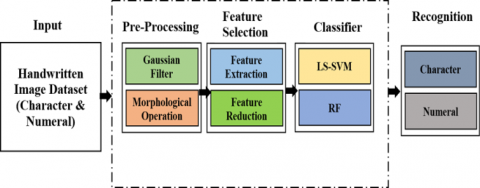Figure 1. Proposed CR model for handwritten character and numeral

The proposed model for handwritten character recognition is illustrated in Figure 1. The proposed model aims to recognize both Odia and Bangala numerals by incorporating two vital stages such as pre-processing (Gaussian filter, morphological operation) and feature selection followed by classification using standard databases of handwritten characters and numerals are collected from various research institutions. The detailed descriptions of each stage are discussed in subsequent paragraph.

3.1 Materials preparation

In the experimental setup, the authors have used the standard database of handwritten Odia character that is taken from NIT, Rourkela . However, for numerals, the authors have also used the database from IIT, BBSR  and NIT, Rourkela . The numeral dataset consists of 10 numerals starting from 0 to 9 and the character set consists of 47 characters as depicted in Figures 2-4, respectively.

## 2.jpg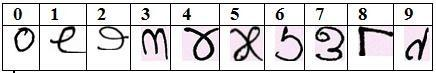Figure 2. Sample of handwritten Odia numeral database from IIT, BBSR

## 3.jpg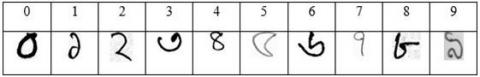Figure 3. Sample of handwritten Bangla numeral database from NIT, RKL

## 4.jpg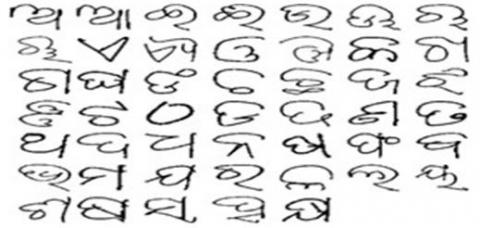Figure 4. Sample of handwritten Odia character database from NIT, RKL

In the above discussed databases, more than 500 samples are listed for the 10 numeral categories and 350 samples are listed for the 47 characters, which were collected from various individuals. Hence, the total input size of handwritten numerals and characters was 500×10 (5000) and 350×47 (16,450) images, respectively.

3.2 Pre-processing

In the OCR system, preprocessing is considered as a crucial step in proposed model, as this state makes the image free from noise and other unwanted features and also improves the quality of the object image. In order to obtain noise-less images, the Gaussian filter selected imperially. In order to report a high percentage of the accuracy for the handwritten recognition model, the authors have also performed dilation morphological operation over the handwritten image to detect the exact location of edges and the boundary line in an Odia character and numerals.

The process of handwritten character recognition involves a well-defined approach for the segmentation and feature extraction. As this process is performed in the Odia character and numerals, it is quite interesting to report the uniqueness of each image. As there are few variations in numbers as compared to characters, there is always ambiguity in the classifier. Different handwritten patterns used by different writers make the recognition problem more complex. In order to find a feasible solution to such problems, the authors must have applied a significant feature section method for each individual character as well as a classic one. This calculates the minimum key values or characteristics, which makes it different from the others. Here, in the proposed model of the handwritten recognition system, the authors have suggested a classical technique named as curvelet analysis . For the proposed experimental setting, selected fast discrete curvelet transformation (FDCT) [36, 37] as our feature extraction technique. Initially, the Fourier transformation was applied, and then the wavelet transformation was used. The wavelet transformation  is considered very efficient in representing 1D object, but it is quite challenging in representing 2D and multi-scale objects such as curve and lines. The basic difference between the curvelet and the wavelet transformation lies in the orientation degree of localization. Mostly, these are outcome based and concentrate on attributes such as resolution and directional selectivity, whereas localization outcomes are affected by the curvelet transformation [39, 40]. This transformation is considered an advanced version of the ridge let transform that is used for representing the curve singularity. The first implantation over the sub- band images led to the first-generation curvelet and latter, the second-generation wavelet was adopted [36, 39]. Generally, it is a block-based approach and very powerful in representing the curve, edges, and texture of the image dataset. From the literature analysis, the authors have observed that the curvelet is quite significant in representing the edges and texture and represents the inner cross-product of a signal f, which can be expressed as follow:

$(i, l, k)=\left\langle f, \lambda_{i,,}\right\rangle$        (1)

In the above equation, λ i, l, k is the generalized curvelet function that holds the respective scale (i), orientation (l) and, position (k) values. In the continuous form, the function is represented by 's' along with θ polar coordinate values and the spatial and frequency component χ and ω. Subsequently, in this proposed model can apply the continuous 2D curvelet transform with the frequency domain containing spatial variable 'z', where the respective images can be decomposed at a certain variation of scales along with the variation of orientations:

$U_{i}(s, \theta)=2^{-3 i / 4}\left(2^{-i} s\right) V\left(2^{|i / 2|} \theta / 2 \pi\right)$       (2)

In each case i>i0, and Ui represents the respective Fourier transform as expressed in the above equation. In addition, W(s) and V(t) are defined as the radial and the other as the angular windows. While evaluating the transformation, certain basics functions are used such as:

$\sum_{t=-\infty}^{\infty} W^{2}\left(2^{i} s\right)=1, s \in(3 / 4,3 / 2)$       (3)

$\sum_{l=-\infty}^{\infty} U^{2}(t-1), t \in(-1 / 2,1 / 2)$         (4)

In this segment, we maintained the window in a smooth, nonnegative condition to a real value based on sÎ(1/2,2) and tÎ(-1,1). The above discussed transformation performed at a certain shifting orientation value of scaling, which is 2j of scaling and it must produce a function of q=(q1,q2).

$\lambda_{i, l, k}(q)=\lambda_{i}\left(R_{\theta_{l}}\left(q-q_{k}^{i, l}\right)\right.$       (5)

In the above equation, $\mathrm{R}_{\theta}$ represents the orthogonal rotation at an angle $\theta$. Here, the rate of orientation is expressed as $\theta_{1}=2 \pi .2^{-[\mathrm{i} / 2]}$, l, l=0,1,2, …, k=(k1,k2)$\in$z2, which indicates the sequence of shift parameters. In addition, the context of the position $\mathrm{q}^{(\mathrm{i}, \mathrm{l})}=\mathrm{R}_{\theta \mathrm{l}}^{-1}\left(\mathrm{k}_{1} \cdot 2^{-\mathrm{i}}, \mathrm{k}_{2} \cdot 2^{-\mathrm{i} / 2}\right)$ and the continuous forms of the curvelet are expressed as follows:

$C_{(i, l, k)}=\frac{1}{(2 \pi)^{2}} \oint \hat{\mathrm{f}}(\omega) \overline{\lambda_{1, j, \mathrm{k}}} \mathrm{dw}$      (6)

From the above-mentioned equation, we can easily retrieve the coefficient of the transformation over the frequency plane as follows:

$C_{(i, l, k)}=\frac{1}{(2 \pi)^{2}} \oint \hat{\mathrm{f}}(\omega) \overline{\lambda_{1, \mathrm{~J}, \mathrm{k}}} \mathrm{dw}$      (7)

Subsequently, we also employed the discrete curvelet transform as $C_{(i, l, k)}^{d}$, which is considered for a Cartesian array of any input images as expressed below:

$C_{(i, l, k)}^{d}=\sum_{0 \leq x_{1}, y_{1}<n} f\left[x_{1}, y_{1}\right] \overline{\lambda_{l, l, k}^{d}\left[x_{1}, y_{1}\right]}$    (8)

This curvelet-based approach exhibits variation in scaling along with the different orientation of the decomposition level of the frequency plane. This curvelet-based approach is generally represented in the wedges at certain scales and orientation, as shown in Figure 5 where the shaded region is the wedge one. In the proposed model, the authors have evaluated the second- order discrete curvelet, known as fast discrete curvelet transform (FDCT), up to the 3rd level of decomposition. In most cases the authors have apply two conditions for evaluating the FDCT: one is unequal space in Fourier transform (USFFT)  and the other is the wrapping method . In order to report the desired feature vector, the authors have applied the 2d- FDCT method in both the datasets of handwritten numerals and characters, which are approximation feature values of the third level decomposition. In addition, the proposed model has applied the wrapping method owing to its robust nature and least redundancy. Here, each image of the character and numeral has been processed by the md number of the highest coefficients of FDCT for each scale and every orientation. The authors have considered N r and N c for rows and column respectively along with S c that is the scale used for FCDT, which is expressed as follows:

$S_{c}=\left\lceil\log _{2}^{\left(\min \left(N_{r}, N_{c}\right)\right)-3}\right\rceil$        (9)

Assume a scenario where an image of a certain size, say 256 *256; for such types of images, the evaluated number of scale will be 5 at various orientations but will be absent for the initial and last scales of the decomposition. However, the authors can also observe variation in the angle of orientation of 16, 32, and 32 for respective scales with values 2, 3, and 4. Here, all sub-bands of images are considered, and first one represents the approximation part and the rest the detail one. All required steps to calculate the feature vectors are well depicted in Algorithm 1. Henceforth, by obtaining such phenomenon, it can easily obtain that the desired feature vector in the matrix format for all handwritten images by applying the wrapping function of the curvelet transform which can be used for further processing in the recognition model.

## 5.png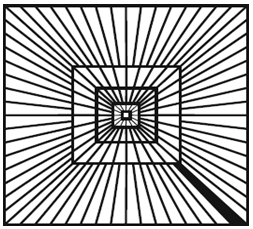Figure 5. Proposed CR model for handwritten character and numeral

Algorithm I: Estimation of Feature Vector using FDCT.

Input: Read the image from database: F[x1,x2];0≤x1,x2x;

Output: Report the curvelet coefficient: Coeff(i,l,k)

1. At each input of F[x1,x2], perform 2d-FDCT and report the coefficients of the Fourier transform as follow:

## 0.png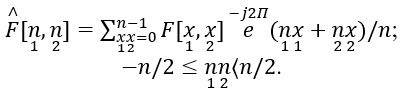1. For every value of scale i and angel l, report the cross-product of $\hat{V j l}\left[\begin{array}{l}n, n \\ 1,2\end{array}\right] \hat{F}\left[\begin{array}{c}n, n \\ 1,2\end{array}\right]$.
2. where, $\hat{V j} l\left[\begin{array}{ll}n & n \\ 1 & 2\end{array}\right]$  represents the discrete value of the window in frequency.
3. Then perform the wrapping as $f_{i, l}^{\approx}\left[\begin{array}{cc}n & n \\ 1 & 2\end{array}\right]$=Z($\hat{V j l}\left[\begin{array}{l}n, n \\ 1,2\end{array}\right] \hat{F}\left[\begin{array}{c}n, n \\ 1,2\end{array}\right]$) .

where, $0 \leq \underset{1}{n}<\underset{1, i}{L}$  and $0 \leq \underset{2}{n}<\underset{2, i}{L}$  for $\theta \in\left(-\Pi / /_{4}, \Pi / 4\right)$  and L1,i, L2,i, represents the length and width of rectangle at scale j.

1. Subsequently, calculate the curvelet coefficient Coeff(i,l,k) by performing 2d Fourier transform to each $f_{i, l}^{\approx}\left[\begin{array}{cc}n & n \\ 1 & 2\end{array}\right]$.

All the relevant datasets of both numerals and characters are followed by applying Algorithm I. Along all the sub-bands it can be assumed that we can obtain ηa which may be represented as Ma where a=1,2,...ηa. We have also noted the values of curvelet at the desired angle θ which is the same as that obtained at Π+θ. Therefore, the desired feature vector obtained for both the dataset images is expressed as follows:

$F_{v e c}=\left[M_{1}^{l_{1} . . l_{c}, M_{2}} l_{1}, \ldots l_{c}, \ldots \ldots \ldots, M_{\eta b}^{l_{1}, \ldots, l_{c}}\right]$       (10)

where, ηb represents the symmetric set of excluded sub-bands and lc represents the highest value of the co-efficient along the ηb sub-band. In the proposed model, all the desired obtained values are processed for further use in phases. All the subsequent steps are followed for the two handwritten database images of characters and numerals one after another, and the reported desired feature vectors are depicted in Figure 6 and 7.

## 6.png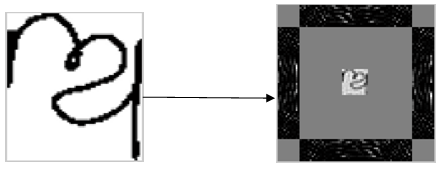Figure 6. Handwritten Odia character and its respective frequency level of discrete curvelet

## 7.png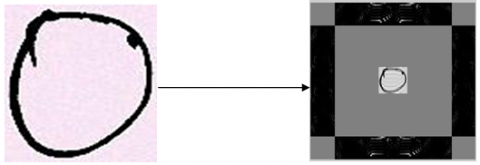Figure 7. Handwritten Odia numeral and its respective frequency level of discrete curvelet

3.4 Reduction of feature vector dimension

The conversion of a higher feature dimension value to a smaller dimension value is known as the reduction analysis over the feature vector set. In this process, the higher dimension of the feature vector produces very high load and higher memory that exhibits poor performance. For avoiding such problems, PCA  is applied for mapping of these high dimensional entities to low dimensional entities, which has been widely used for reducing the dimensionality of the feature vector. PCA generally exhibits the orthogonal transformation of the input data and reports the achieved matrices of different variances, which helps in predicting the score values known as PC score or principal components. In addition to PCA, in this step, the linear discriminate analysis (LDA) is also introduced for performing the reduction in dimension. This discriminate analysis always produces a low number of feature spaces and reports the variation among classes; therefore, this analysis is widely used by researchers. This supervised-based approach provides a sample that very different from other classes but similar to the same class. Generally, the LDA discriminates the classes and also exhibits low performance for higher dataset constraints in order to obtain small sample size . A suitable solution is proposed for higher in dimensions but for small sample sizes. For instance, consider a scatter matrix termed as $S_{\text {mat }}$ which is singular. Then, calculate the value of m+n, where m all total classes available and n is the required vector dimension. Generally, it is nearly impossible to obtain $S_{\text {mat }}$ in a singular form, so a hybrid approach termed as PCA is used along with LDA. In the cases of PCA+LDA, initially M dimensions are reduced to N dimensions with the help of PCA and thereafter they are reduced to ddimensions by harnessing LDA such that $\mathrm{d} \ll \mathrm{N} \ll \mathrm{M}$.

Before processing the PCA with LDA, a certain necessary action is taken. In addition, we must also ensure that the desired feature vector must be normalized one with zero mean and unit variance. This particular step must be followed so that an optimal feasible solution for the feature vector can be suggested. All the eigenvalues are arranged according to their decreasing values and the normalized cumulative sum of variance (NCSV) should also be reported in that phase.

$\operatorname{NCSV}(i)=\frac{\sum_{u=1}^{\alpha} \alpha(u)}{\sum_{u=1}^{D} \alpha(u)} 1 \leq i \leq D$       (11)

The eigenvalues of the images are denoted by α(u) and the respective dimension of the feature set is represented by D. In order to make the subsequent step successful, certain threshold values are maintained manually so that the desired relevant reduced feature vectors with suitable dimensions are considered to achieve high recognition accuracy for handwritten characters and numerals, which are termed as the basic vectors. All the essential steps are discussed below in Algorithm II.

Algorithm. II: Feature reduction using PCA+LDA.

Require: Feature matrix: FM of size N*D

Ensure: Reduced feature matrix: Fr of size N*L

Functions PCA () and LDA () reduce the dimensions by using PCA and LDA, respectively.

1: Choose a dimension M $\triangleleft$ Reduced dimension using the PCA

2: F’(N *M) PCA(FM; M)

3: Select a dimension L using the NCSV measure $\triangleleft$ Reduced dimension using the LDA

4: Fr(N * L) LDA(F’;L)

5: Output the reduced matrix Fr

3.5 Classification by the LS-SVM, RF classifiers

In the OCR system, the classification stage  is considered the most important stage in a model. It defines the robustness of the proposed model with respect to the variation in the datasets. For the classification analysis, the proposed model have employed the support vector machine (LS-SVM) and random forest (RF) as classifiers. Subsequently, the obtained basic feature sets of datasets are processed as input parameters along with the class levels of the above- mentioned two classifiers one by one. All the extracted key feature data were processed through classification implemented by using LS-SVM classifier  and followed by the RF classifier . This process is not that much effective in the case of larger datasets, and sometime leads to complex networks, so for removing this drawback LS-SVM is used in this paper. It has been observed that LS-SVM exhibits more incremental responses as compared to the traditional ones in terms of the addition of some equality constraints. Generally, an optimization analysis is applied for solving the linier solution to the problem domain. The performance of the LS-SVM classifier can be expressed as follows:

$\min _{c, d, e_{r}} j\left(c, d, e_{r}\right)=\frac{1}{2} c^{T} c+D \frac{1}{2} \sum_{i=1}^{N} e_{r_{i}}^{2}$       (12)

With some constraints as

$Z_{i}\left[c^{T} \phi(p i)+d\right]=1-e_{r}, i=1,2,3, \ldots, N$         (13)

Here {pi,Zi} is the obtained key feature vector that acts as a training set of N samples with pi data. ϕ(.) and c represent the mapping function and associated weight, respectively. D>0, d and er are used as the regularization factor, bias, and error, respectively. In addition, we also included the three-kernel function such as linear, polynomial, and radial basis functions, to the LS-SVM. The required solution for LS-SVM achieves the optimality by the help of the Lagrangian method, which is expressed as follows:

$\mathrm{L}\left(\mathrm{c}, \mathrm{d}, \mathrm{e}_{\mathrm{r}}, \lambda\right)=\mathrm{j}\left(\mathrm{c}, \mathrm{d}, \mathrm{e}_{\mathrm{r}}\right)-\sum_{\mathrm{i}-1}^{\mathrm{N}} \lambda_{\mathrm{i}}\left\{\mathrm{y}_{\mathrm{i}}\left[\mathrm{c}^{\mathrm{T}} \phi\left(\mathrm{p}_{\mathrm{i}}+\right.\right.\right.$

$\left.\mathrm{d})]-1+\mathrm{e}_{\mathrm{ri}}\right\}$       (14)

In the same process, some constraints or kernel functions are harnessed over the dataset to report the actual class level of each individual handwritten image. We also executed the polynomial kernel in addition to the other two kernels, namely, linear and radial basics function (RBF). Apart from LS-SVM, another random forest (RF) classifier  was analyzed for the handwritten characters and numerals. It was found to be quite simpler and flexible in nature, so it was considered to be very helpful for large datasets. Basically, RF is a well-defined approach of tree which follows the decision-based structured classifier and is completely based on random samples that have uniform distribution among trees. Hence, it is considered to be the most positive point of this method and works or proceeds with the tree in the depth wise location. In this random-based approach, each tree is structured considering the values of random vectors and sampled with the same distribution using the forest classifier . Suppose the random forest represents the methodology for building L tree for classification then it is denoted by $\left\{\mathrm{T}_{\mathrm{j}}\left(\mathrm{X}, \theta_{\mathrm{j}}\right\}\right.$. In this tree, X is the input feature and $\theta_{\mathrm{j}}=\left\{\theta_{1}, \theta_{2}, \ldots, \theta_{\mathrm{j}}\right\}$  is the respective random vector for the jth  tree with a training size of N.  Apart from this, $\theta_{1}, \theta_{2}, \ldots, \theta_{\mathrm{j}}$ are the integral values drawn from the random uniform distribution. Therefore, it creates a subspace method $\theta_{\mathrm{j}}$ that provides K number of integers with an interval M. For any given sample x, the chances of being classified by T are denoted by a probability function like $P\left(\frac{C}{x}, T\right)$. Moreover, it is verified that if the sample x belongs to class $C(C \in\{1,2, \ldots, C\}$, it is represented as follows:

$P\left(\frac{C}{x}, T\right)=\frac{n(c)}{n}$       (15)

In this case, $\mathrm{n}(\mathrm{c})$ is the desired sample taken from the training sample, which makes them to fall in v(x). Apart from this, all numbers in the training sample are made to fall in v(x). At a certain step, if an unknown sample is classified then the equation is altered to belong to L numbers of trees as follows:

$\mathrm{P}\left(\frac{\mathrm{C}}{\mathrm{x}}, \mathrm{T}\right)=\frac{1}{\mathrm{~L}} \sum_{\mathrm{i}=1}^{\mathrm{L}} \mathrm{P}\left(\frac{\mathrm{C}}{\mathrm{x}}, \mathrm{T}_{\mathrm{i}}\right)$       (16)

4. Implementation of the Proposed Model

In the proposed model of a recognition system, the authors have evaluated each step in detail. All the data images of both characters and numerals are processed with the subsequent phases of the model one by one. In order to predict the robustness of the model, divided the above process into two halves, namely, FCDT+PCA+LDA+LS-SVM and FCDT+PCA+LDA+RF. Initially, the dataset is spilt into training and testing phases in ration of 70:30, and in the next phase if new data appear then the classification of the new data object is performed. All the above-mentioned steps are encoded in Algorithm III as shown below.

Algorithm. II: Feature reduction using PCA+LDA.

Input: Read the image from the database

Output: Recognition Accuracy

Step 1: Perform image acquisition of the handwritten characters and numerals from datasets;

Step 2: Process the data image according to the pre-processing stage and remove the noises associated with data images;

Step 3: The entire processed image is followed in the next feature evaluation section, where the fast discrete curvelet transformation (FDCT) is performed up to 3rd level and the desired feature vector is listed;

Step 4: Therefore, the feature reduction through PCA+LDA, that is, from D to d, is evaluated, which provides the normalized cumulative sum of variance (NCSV). Thus, the d basis vector is retained as the primary key feature;

Step 5: The basis vectors are processed to train and test the two selected classifiers;

Step 6: All the vector coefficients are input into the LS-SVM and RF classifiers one by one. In addition, the training of the classifier is performed and subsequently, class levels of the input data image are reported;

End the algorithm when classification processed.

5. Analysis of Simulation Results

In order to successfully implement the recognition system, the authors have applied the proposed algorithms I and II to the standard dataset of handwritten characters and numerals. Similarly it can show at third level decomposition of the discrete curvelet transformation (FCDT) in the feature extraction section. Moreover, the approximation feature vector along the sub-bands of the decomposition level of images is reported in 21*21 numbers of features in this transformation. As already discussed, higher dimensions are again processed for the next segment, that is, the dimension reduction. In order to obtain better efficiency or to make the recognition process easier, it is required to perform a reduction in the dimension vector reported from the FCDT. Hence, PCA+LDA is processed over the feature set and it produces some basic vectors that represent the reduced dimension in the feature vector in terms of NCVS. In addition, the proposed model has maintained the threshold value at 0.9 for the recognition system. In the simulation part, it has been considered 350*47 numbers of handwritten character images and 500*10 numbers of handwritten numeral images. After performing the reduction to the feature vector by using PCA+LDA, the authors have reported 50 and 60 numbers of primary feature vectors which shows the reduction in dimension of the feature vector for handwritten characters and handwritten numerals, and act as inputs to LS-SVM and RF classifiers, respectively. Here, it has also noted the recognition rate at different ranges of features. All the well-defined steps are listed in Algorithm III. The authors have performed the classification over three datasets. In this process, first all the characters are classified and then they are followed by handwritten numerals.

6. Research Discussion

## 8.png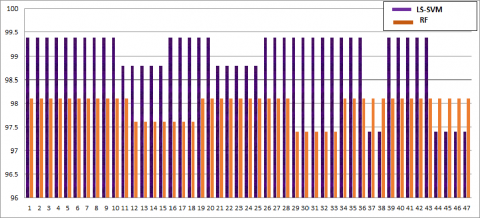Figure 8. Comparison between LS-SVM & RF classifier used for handwritten Odia character

## 9.pngFigure 9. Comparison between LS-SVM & RF classifier used for handwritten Odia numerals

## 10.pngFigure 10. Comparison between LS-SVM, RF & CNN classifier used for handwritten Bangla numerals

In this proposed model to have high recognition the primary focus is in feature extraction section. In order to handle the invariant nature of handwritten image, here fast discrete curvelet transformation (FDCT) is harnessed over the image. This feature vector coefficient scaled with the parameters as orientation, location of the pixel and due its precise sparse representation of object along with edges for that widely used. As this proposed model include handwritten data images, where mostly the Odia and Bangla characters are very much identical in terms of orientation, shape, size. So the curvature analysis of the image has been reported in this proposed model. Apart from it to make the system less overhead here dimension optimality has been reported through PCA, LDA. By harnessing these linear transformations over to the feature set to report least feature subspace. In order to make the system high robust the prosed system has also evaluated the make over all analysis among these feature sets. As the outcome of the simulation analysis, the proposed model produces very promising high recognition rates for individual writers. In the proposed model have applied classifier namely as LS-SVM, RF classifiers for Odia handwritten characters, Odia and Bangla numerals. 99.01%, 98.1% for Odia character, 98.6%, 97.6% for Odia numerals and 97.6%, 96.3% for Bangla numerals by using the LS-SVM and RF classifiers Apart from this, the authors have also provided a comparison of both the classifiers for both the datasets as depicted in Figure 8, 9, and 10 respectively.

7. Conclusions

This proposed model primarily focused on the variant features of the handwritten images and provided an automated system for the recognition of the numerals and characters. All the essential steps, such as acquisition, preprocessing, and proper feature extraction methods, and well-defined classifiers were used to make the recognition system successful. In order to make the system robust, we trained the system with a wide range of styles of handwritten images that have been reported here as a standard database. To make the system less complex, in proposed model have performed a reduction in the dimension of the feature vector. Hence, PCA and LDA are harnessed over the feature set in order to produce the reduced version of the feature vector and to be considered as the primary key feature vector. Apart from this, the proposed model promoted three classifiers, namely, LS-SVM and RF Classifiers, in the segment of the classification phase. Initially, the recognition is performed by the LS-SVM classifier and subsequently, followed by the RF classifier. As an outcome of the proposed model, the overall accuracy of handwritten Odia character datasets is reported as 99.01%, 98.1% respectively. Similarly for Odia handwritten numerals 98.6% and 97.6%, respectively and for Bangla numerals 97.6%, 96.3% recognition rate is achieved. From the analysis of results, the proposed models have concluded that LS-SVM performs better as compared to RF based on our problem domain. Proposed model outperforms better as compared to the state-of-the-art methods in terms of both aspect of recognition accuracy and using least number of optimal features are depicted in Table 2 and Table 3 respectively.

Table 2. Analysis over certain well-defined approach for handwritten Odia characters

 Reference No. and Author Name Handwritten Dataset Used Techniques used for Feature Extraction Classifier Used Reported Recognition rate (%) Pal et al.  ISI Kolkata Handwritten Odia Characters Curvature Feature values Modified Quadratic Classifier 94.6 Bhomik et al.  ISI Kolkata Handwritten Odia Characters Stroke calculation along Horizontal and Vertical Neural Network (NN) 95.89, 90.50 Sethy et al.  NIT, RKL Handwritten Odia Characters DWT, PCA BPNN 94.8 Mohapatra et al.  NIT, RKL HandwrittenOdia Characters Stockwell Transform +PCA ANN 98.55 Mishra et al.  NIT, RKL HandwrittenOdia Characters DCT and DWT SVM 92, 87.5 Das et al.  Bangla Character CMATERdb2.1.2database H-Word Net H-Word Net 96.1 Das et al.  OHCSv1.0 MJCN RF BPNN 97.4 95.64 Proposed Model NIT RKL Odia Characters FDCT+LDA+PCA LS-SVM RF 99.01 98.1

Table 3. Analysis over certain well-defined approach for handwritten numerals

 Reference No. and Author Name Handwritten Dataset Used Techniques used for Feature Extraction Classifier Used Reported Recognition rate (%) Bhomik et al.  ISI Kolkata Handwritten Odia numerals Horizontal, Vertical strokes HMM 95.89, 90.50 Sethy et al.  ISI Kolkata Handwritten Odia Numerals Binarization and DCT BPNN 80.2, 90 Das et al.  NIT, RKL Bangla handwritten Numerals ISI Kolkata Handwritten Odia Numerals ELM ELM ELM ELM 96 96.5 Sarangi et al.  ISI Kolkata Handwritten Odia numerals Pattern Matching HNN 95.4 Das et al.  NIT, RKL Bangla handwritten Numerals MJCN RF 95.1 BPNN 90.5 Proposed Model IIT, BBS handwritten Numerals FDCT+LDA+PCA LS-SVM 98.6 RF 97.6 NIT, RKL Bangla handwritten Numerals FDCT+LDA+PCA LS-SVM 97.6 RF 96.3
Acknowledgment

In this proposed model the authors are very much thankful to the NIT, RKL and IIT, BBS. The authors are sincerely grateful towards to the Computer Science Engineering department, CET BBSR and also highly appreciate to all authors of the reference.

References

 Mantas, J. (1986). An overview of character recognition methodologies. Pattern Recognition, 19(6): 425-430. https://doi.org/10.1016/0031-3203(86)90040-3

 Govindan, V.K., Shivaprasad, A.P. (1990). Character recognition-A review. Pattern Recognition, 23(7): 671-683. https://doi.org/10.1016/0031-3203(90)90091-X

 Pal, U., Chaudhuri, B.B. (2004). Indian script character recognition: A survey. Pattern Recognition, 37(9): 1887-1899. https://doi.org/10.1016/j.patcog.2004.02.003

 Patra, P.K., Nayak, M., Nayak, S.K., Gobbak, N.K. (2002). Probabilistic neural network for pattern classification. In Proceedings of the 2002 International Joint Conference on Neural Networks. IJCNN'02 (Cat. No. 02CH37290), 2: 1200-1205. https://doi.org/10.1109/IJCNN.2002.1007665

  Saeys, Y., Inza, I., Larranaga, P. (2007). A review of feature selection techniques in bioinformatics. Bioinformatics, 23(19): 2507-2517. https://doi.org/10.1093/bioinformatics/btm344

 Ma, S., Kosorok, M.R. (2009). Identification of differential gene pathways with principal component analysis. Bioinformatics, 25(7): 882-889. https://doi.org/10.1093/bioinformatics/btp085

 Kuhnke, K., Simoncini, L., Kovacs-V, Z.M. (1995). A system for machine-written and hand-written character distinction. In Proceedings of 3rd International Conference on Document Analysis and Recognition, 2: 811-814. https://doi.org/10.1109/ICDAR.1995.602025

 Pal, U., Wakabayashi, T., Kimura, F. (2007). A system for off-line Oriya handwritten character recognition using curvature feature. In 10th International Conference on Information Technology (ICIT 2007), pp. 227-229. https://doi.org/10.1109/ICIT.2007.63

 Bhowmik, T.K., Parui, S.K., Bhattacharya, U., Shaw, B. (2006). An HMM based recognition scheme for handwritten Oriya numerals. In 9th International Conference on Information Technology (ICIT'06), pp. 105-110. https://doi.org/10.1109/ICIT.2006.29

 Chanda, S., Franke, K., Pal, U. (2012). Text independent writer identification for Oriya script. In 2012 10th IAPR International Workshop on Document Analysis Systems, pp. 369-373. https://doi.org/10.1109/DAS.2012.86

 Pujari, A.K., Mitra, C., Mishra, S. (2014). A new parallel thinning algorithm with stroke correction for Odia characters. In Advanced Computing, Networking and Informatics, 1: 413-419. https://doi.org/10.1007/978-3-319-07353-8_48

 Mitra, C., Pujari, A.K. (2013). Directional decomposition for Odia character recognition. In: Prasath, R., Kathirvalavakumar, T. (eds) Mining Intelligence and Knowledge Exploration. Lecture Notes in Computer Science, vol 8284. Springer, Cham. https://doi.org/10.1007/978-3-319-03844-5_28

 Nayak, M., Nayak, A.K. (2018). Recognition of Odia conjunct characters using a hybrid ANN-DE classification technique. In Progress in Advanced Computing and Intelligent Engineering, pp. 71-79. https://doi.org/10.1007/978-981-10-6875-1_8

 Kumar, B., Kumar, N., Palai, C., Jena, P.K., Chattopadhyay, S. (2013). Optical character recognition using ant miner algorithm: A case study on Oriya character recognition. International Journal of Computer Applications, 61(3): 17-22

 Dash, K.S., Puhan, N.B., Panda, G. (2015). Handwritten numeral recognition using non-redundant Stockwell transform and bio-inspired optimal zoning. IET Image Processing, 9(10): 874-882.

 Dash, K.S., Puhan, N.B., Panda, G. (2014). A hybrid feature and discriminant classifier for high accuracy handwritten Odia numeral recognition. In 2014 IEEE Region 10 Symposium, pp. 531-535. https://doi.org/10.1109/TENCONSpring.2014.6863091

 Pujari, P., Majhi, B. (2015). Notice of Removal: A comparative study of classifiers on recognition of offline handwritten Odia numerals. In 2015 International Conference on Electrical, Electronics, Signals, Communication and Optimization (EESCO), pp. 1-5. https://doi.org/10.1109/EESCO.2015.7253699

 Dash, K.S., Puhan, N.B., Panda, G. (2015). On extraction of features for handwritten Odia numeral recognition in transformed domain. In 2015 Eighth International Conference on Advances in Pattern Recognition (ICAPR), pp. 1-6. https://doi.org/10.1109/ICAPR.2015.7050694

 Sethy, A., Patra, P.K. (2016). Off-line Odia handwritten numeral recognition using neural network: a comparative analysis. In 2016 International Conference on Computing, Communication and Automation (ICCCA), pp. 1099-1103. https://doi.org/10.1109/CCAA.2016.7813880

 Sethy, A., Patra, P.K., Nayak, D.R. (2018). Off-line handwritten Odia character recognition using DWT and PCA. In Progress in Advanced Computing and Intelligent Engineering, 187-195. https://doi.org/10.1007/978-981-10-6872-0_18

 Mohapatra, R.K., Majhi, B., Jena, S.K. (2015). Classification of handwritten Odia basic character using Stockwell transform. International Journal of Applied Pattern Recognition, 2(3): 235-254.

 Sarangi, P.K., Sahoo, A.K., Ahmed, P. (2012). Recognition of isolated handwritten Oriya numerals using Hopfield neural network. International Journal of Computer Applications, 40(8): 36-42.

 Das, N., Pramanik, S. (2019). Recognition of handwritten Bangla basic character and digit using convex hall basic feature. In 2009 International Conference on Artificial Intelligence and Pattern Recognition (AIPR-09).

 Rabby, A.K.M., Islam, M., Hasan, N., Nahar, J., Rahman, F. (2020). Borno: Bangla handwritten character recognition using a multiclass convolutional neural network. In Proceedings of the Future Technologies Conference, pp. 457-472. https://doi.org/10.1007/978-3-030-63128-4_35

 Bora, M.B., Daimary, D., Amitab, K., Kandar, D. (2020). Handwritten character recognition from images using CNN-ECOC. Procedia Computer Science, 167: 2403-2409. https://doi.org/10.1016/j.procs.2020.03.293

 Das, D., Nayak, D.R., Dash, R., Majhi, B., Zhang, Y.D. (2020). H-WordNet: A holistic convolutional neural network approach for handwritten word recognition. IET Image Processing, 14(9): 1794-1805.

 Sethy, A., Patra, P.K., Nayak, S.R. (2020). Offline handwritten numeral recognition using convolution neural network. Machine Vision Inspection Systems: Image Processing, Concepts, Methodologies and Applications, 1: 197-212. https://doi.org/10.1002/9781119682042.ch9

 Das, D., Nayak, D.R., Dash, R., Majhi, B. (2020). MJCN: Multi-objective Jaya Convolutional Network for handwritten optical character recognition. Multimedia Tools and Applications, 79(43): 33023-33042. https://doi.org/10.1007/s11042-020-09457-6

 Kessentini, Y., Paquet, T., Hamadou, A.B. (2010). Off-line handwritten word recognition using multi-stream hidden Markov models. Pattern Recognition Letters, 31(1): 60-70. https://doi.org/10.1016/j.patrec.2009.08.009

 Thomas, S., Chatelain, C., Heutte, L., Paquet, T., Kessentini, Y. (2015). A deep HMM model for multiple keywords spotting in handwritten documents. Pattern Analysis and Applications, 18(4): 1003-1015. https://doi.org/10.1007/s10044-014-0433-3

 Cheikhrouhou, A., Kessentini, Y., Kanoun, S. (2020). Hybrid HMM/BLSTM system for multi-script keyword spotting in printed and handwritten documents with identification stage. Neural Computing and Applications, 32(13): 9201-9215. https://doi.org/10.1007/s00521-019-04429-w

 Kessentini, Y., BenAbderrahim, S., Djeddi, C. (2018). Evidential combination of SVM classifiers for writer recognition. Neurocomputing, 313: 1-13. https://doi.org/10.1016/j.neucom.2018.05.096

 Jemni, S.K., Kessentini, Y., Kanoun, S. (2020). Improving recurrent neural networks for offline Arabic handwriting recognition by combining different language models. International Journal of Pattern Recognition and Artificial Intelligence, 34(12): 2052007. https://doi.org/10.1142/S0218001420520072

 Mishra, T.K., Majhi, B., Sa, P.K., Panda, S. (2014). Model based Odia numeral recognition using fuzzy aggregated features. Frontiers of Computer Science, 8(6): 916-922. https://doi.org/10.1007/s11704-014-3354-9

 Das, D., Nayak, D.R., Dash, R., Majhi, B. (2019). An empirical evaluation of extreme learning machine: application to handwritten character recognition. Multimedia Tools and Applications, 78(14): 19495-19523. https://doi.org/10.1007/s11042-019-7330-0

 Candes, E., Demanet, L., Donoho, D., Ying, L. (2006). Fast discrete curvelet transforms. Multiscale Modeling & Simulation, 5(3): 861-899. https://doi.org/10.1137/05064182X

 Candès, E.J. (2003). What is... a curvelet? Notices of the American Mathematical Society, 50(11): 1402-1403.

 Candès, E., Donoho, D. (2000). A surprisingly effective non adaptive representation for objects with edges. Curves and Surfaces.

 Chaplot, S., Patnaik, L.M., Jagannathan, N.R. (2006). Classification of magnetic resonance brain images using wavelets as input to support vector machine and neural network. Biomedical Signal Processing and Control, 1(1): 86-92. https://doi.org/10.1016/j.bspc.2006.05.002

 El-Dahshan, E.S.A., Mohsen, H.M., Revett, K., Salem, A.B.M. (2014). Computer-aided diagnosis of human brain tumor through MRI: A survey and a new algorithm. Expert systems with Applications, 41(11): 5526-5545. https://doi.org/10.1016/j.eswa.2014.01.021

 Xu, B., Ye, Y., Nie, L. (2012). An improved random forest classifier for image classification. In 2012 IEEE International Conference on Information and Automation, pp. 795-800. https://doi.org/10.1109/ICInfA.2012.6246927

 Yang, J., Yang, J.Y. (2003). Why can LDA be performed in PCA transformed space? Pattern Recognition, 36(2): 563-566. https://doi.org/10.1016/S0031-3203(02)00048-1

 Cheikhrouhou, A., Kessentini, Y., Kanoun, S. (2020). Hybrid HMM/BLSTM system for multi-script keyword spotting in printed and handwritten documents with identification stage. Neural Computing and Applications, 32(13): 9201-9215. https://doi.org/10.1007/s00521-019-04429-w

 Kessentini, Y., BenAbderrahim, S., Djeddi, C. (2018). Evidential combination of SVM classifiers for writer recognition. Neurocomputing, 313: 1-13. https://doi.org/10.1016/j.neucom.2018.05.096

 Candes, E., Demanet, L., Donoho, D., Ying, L. (2006). Fast discrete curvelet transforms. Multiscale Modeling & Simulation, 5(3): 861-899. https://doi.org/10.1137/05064182X